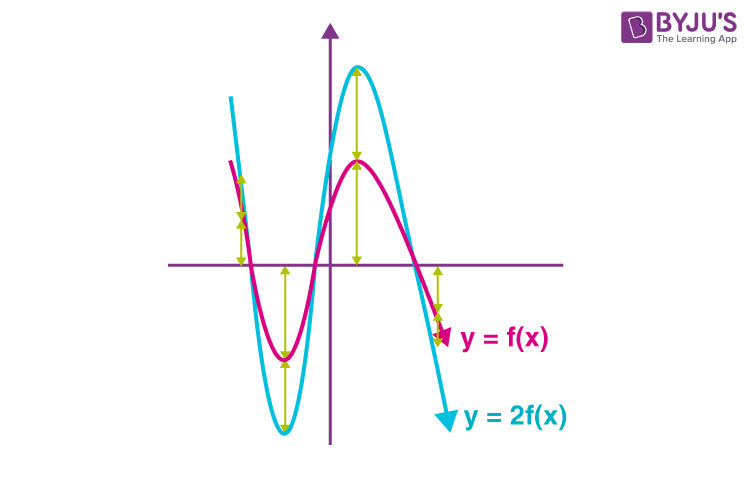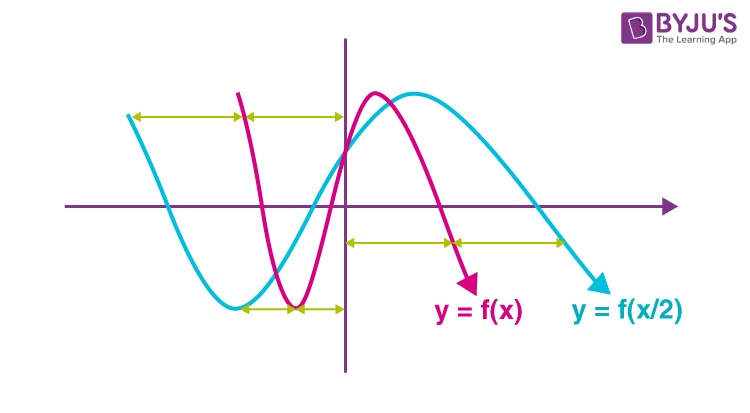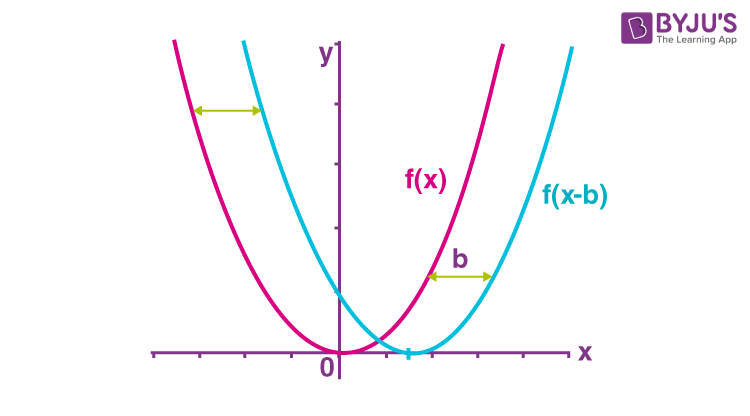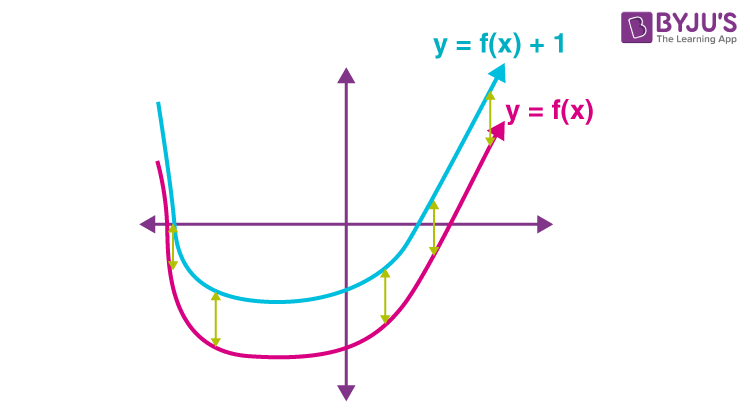# Graph Transformations

Graph transformation is the process by which a graph is modified to give a variation of the proceeding graph. The graphs can be translated or moved about the xy-plane. They can also be stretched, or a combination of these transformations. In this article, we discuss the different graph transformations.

### Stretching of Graphs

If we multiply a function or its independent variable by a constant factor, we can introduce a stretch in its graph. The stretch can be vertical or horizontal. Let us explain horizontal and vertical stretching.

Vertical Stretching

Consider we have to stretch a graph vertically by a factor of 2. Then adjust the y value. Every point of the form (x,y) in function F now moves to the point (x, 2y) in function F*. Similarly, we can stretch a function vertically by c, by multiplying on the outside of the function. cf(x) is the graph of f(x) stretched vertically by c units. The graph given below shows y = f(x) and y = 2f(x).Horizontal Stretching

If we have to stretch a graph horizontally by a factor of 2, then adjust the x value. Every point of the form (x,y) in function F now moves to the point (2x, y) in function F*. Similarly, if we want to stretch a function horizontally by c, we divide on the inside of the function. f(x/c) is the graph of f(x) stretched horizontally by c units. The graph given below gives you a clear idea about horizontal stretching### Translation of Graphs

Horizontal Translation

Horizontally translating a graph means shifting the base graph left or right in the direction of the x-axis. Consider we have to move a graph horizontally by 1 unit, then we should adjust the x value. Every point of the form (x,y) in function f now moves to the point (x+1, y) in function f*. So we can conclude that to move a function right or left, we subtract to the inside of the function. The graph of f(x) shifted right by b units is given by f(x-b).

The value of b determines the direction of the shift. If b < 0, the base graph shifts b units to the left. If b > 0, the base graph shifts b units to the right. The graph given below gives you a clear idea about horizontal translation.Vertical Translation

Vertically translating a graph means shifting the base graph up or down in the direction of the y-axis. A graph is translated b units vertically by moving each point on the graph b units vertically. Consider we have to move a graph up vertically by 1 unit, we should adjust the y value. Every point of the form (x,y) in function f now moves to the point (x, y + 1) in function f*. We can conclude that if we have to move a function up or down, we add to the outside of the function. The graph of f(x) shifted up by b units is given by f(x) + b.

The value of b determines the direction of the shift. if b > 0, the base graph shifts b units upward, and if b < 0, the base graph shifts b units downward. The graph given below makes you clear about vertical translation### Solved Examples

Example 1:

Let the graph y = g(x) has a minimum point at (1,2). Find the minimum point of the graph y = g(x- 3) – 4.

(a) (1, 2)

(b) (4, 2)

(c) (4, -2)

(d) none of these

Solution:

Given y = g(x) has a minimum point at (1, 2).

g(x-3)-4 is a translation of the given graph to the right side by 3 and downwards by 4.

So the point (1, 2) moves to (1+3, 2-4) = (4, -2).

Now the minimum point is (4, -2).

Example 2:

Find the equation of the resulting graph, if we move y = x2+4x-3 to the right side by 3 units and downwards by 2 units.

Solution:

Let f(x) = x2+4x-3

We can rewrite the equation using completing the square method.

f(x) = (x+2)2-7

y+2 = f(x-3)

= (x-1)2-7

= x2-2x-8

x2-2x-8 is the required equation.

Related videos